Question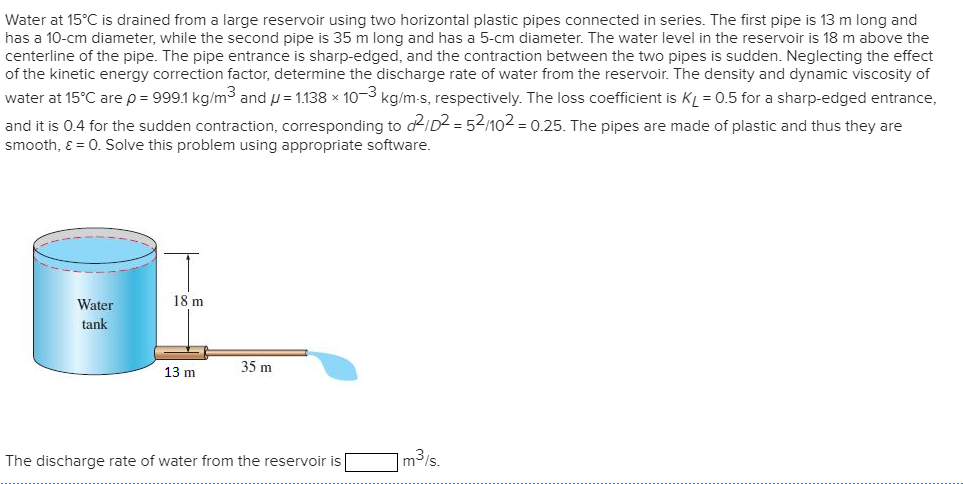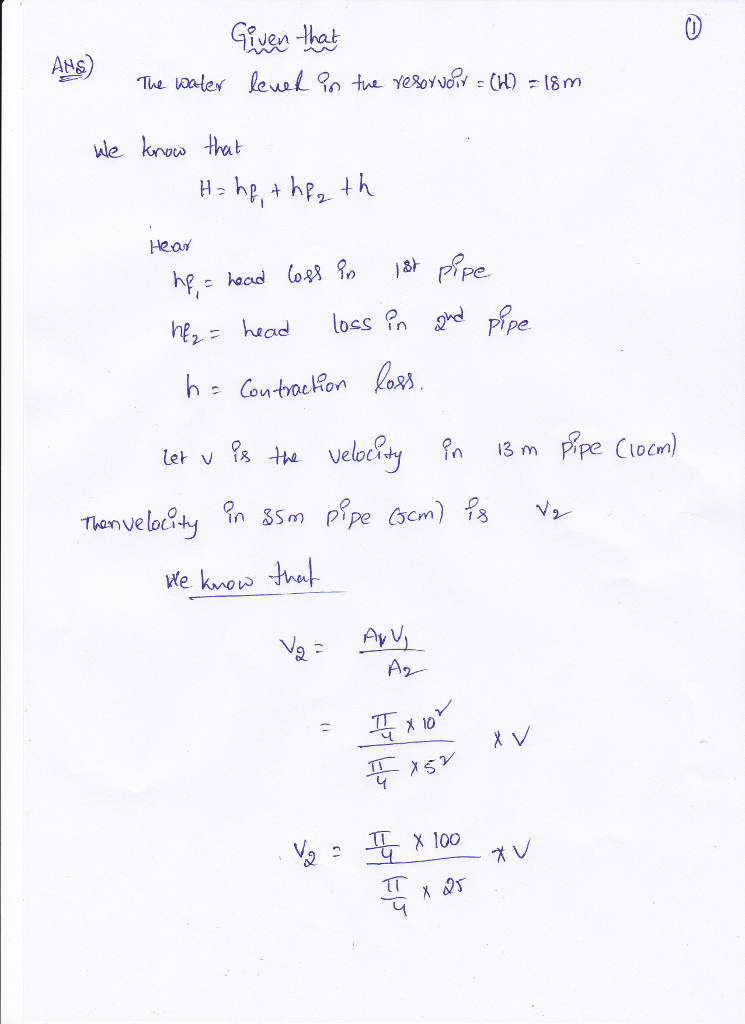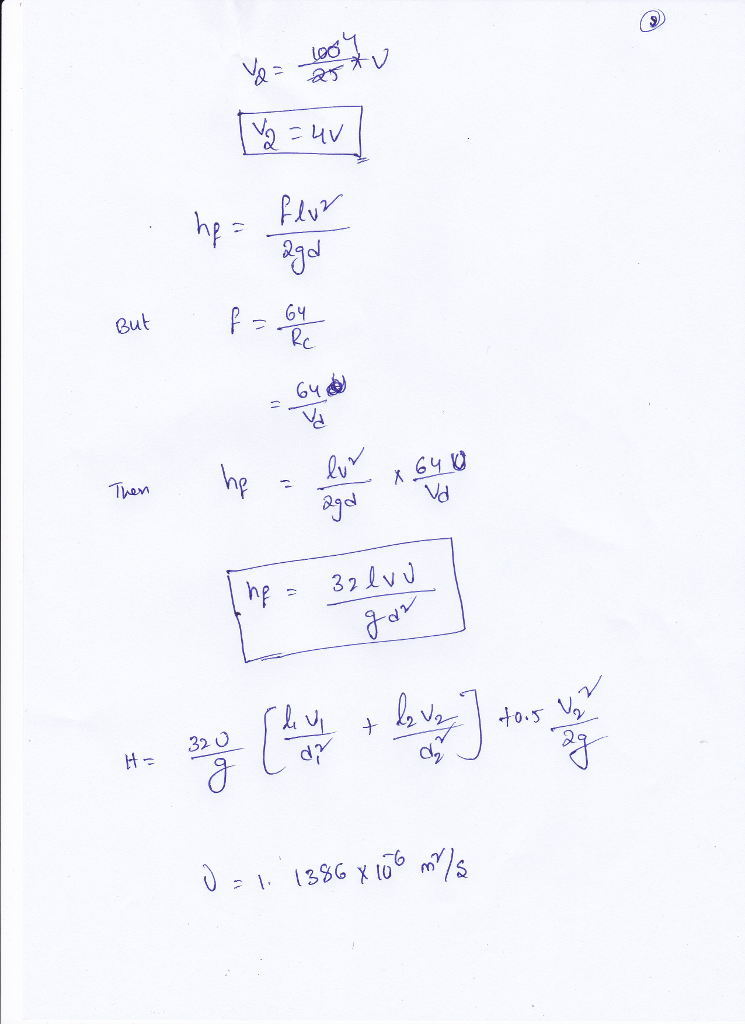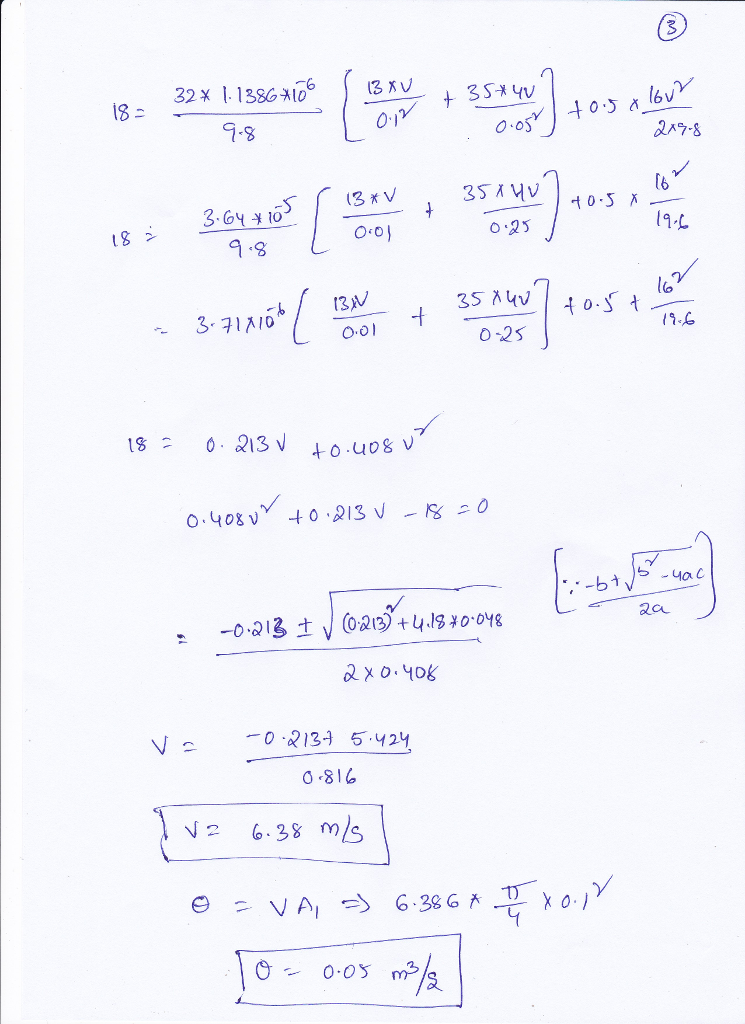#### Earn Coins

Coins can be redeemed for fabulous gifts.

Similar Homework Help Questions
• ### Water is slowly drained from a large reservoir using two horizontal plastic pipes connected in series....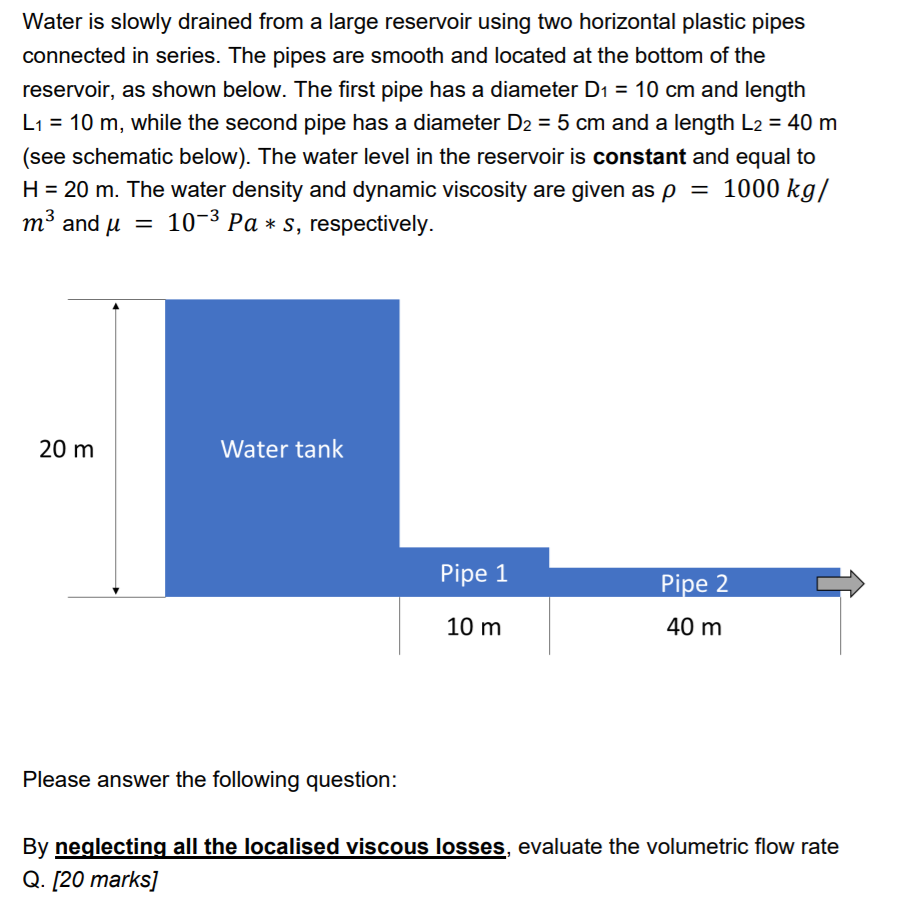Water is slowly drained from a large reservoir using two horizontal plastic pipes connected in series. The pipes are smooth and located at the bottom of the reservoir, as shown below. The first pipe has a diameter D1 = 10 cm and length L1 = 10 m, while the second pipe has a diameter D2 = 5 cm and a length L2 = 40 m (see schematic below). The water level in the reservoir is constant and equal to H...

• ### fluid mechanics ASAP please Problem 4 (50 points) Water is discharged from a reservoir at a rate of 18.103 m3/s using two horizontal cast iron pipes connected in series and a pump between them. T...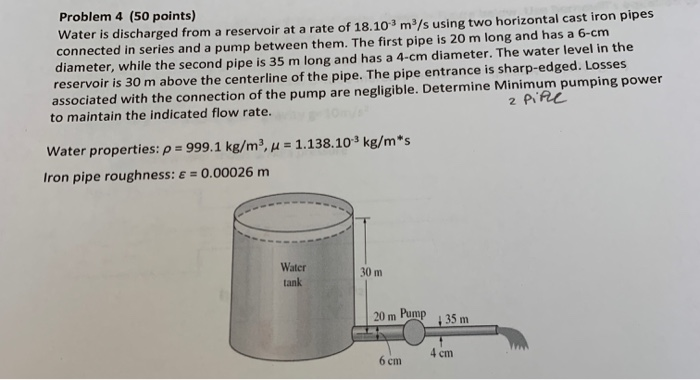fluid mechanics ASAP please Problem 4 (50 points) Water is discharged from a reservoir at a rate of 18.103 m3/s using two horizontal cast iron pipes connected in series and a pump between them. The first pipe is 20 m long and has a 6-cm diameter, while the second pipe is 35 m long and has a 4-cm diameter. The water level in the reservoir is 30 m above the centerline of the pipe. The pipe entrance is sharp-edged. Losses...

• ### Problem 4 (50 points) water is discharged from a reservoir at a rate of 18.103 m2/s using two horizontal cast iron pipes nected in series and a pump between them. The first pipe is 20 m long and...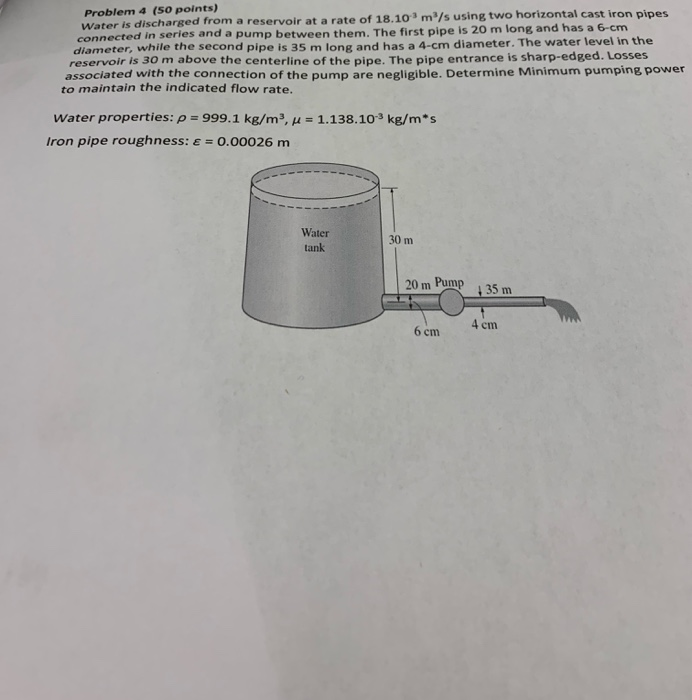Problem 4 (50 points) water is discharged from a reservoir at a rate of 18.103 m2/s using two horizontal cast iron pipes nected in series and a pump between them. The first pipe is 20 m long and has a 6-cm diameter, while the second pipe is 35 m long and has a 4-cm diameter. The water level in the reservoir is 30 m above the centerline of the pipe. The pipe entrance is sharp-edged. Losses associated with the connection...

• ### Water (density = 1000 kg/m3, viscosity = 1.15 x 10-3 N-s/m2) is delivered from a large reservoir ...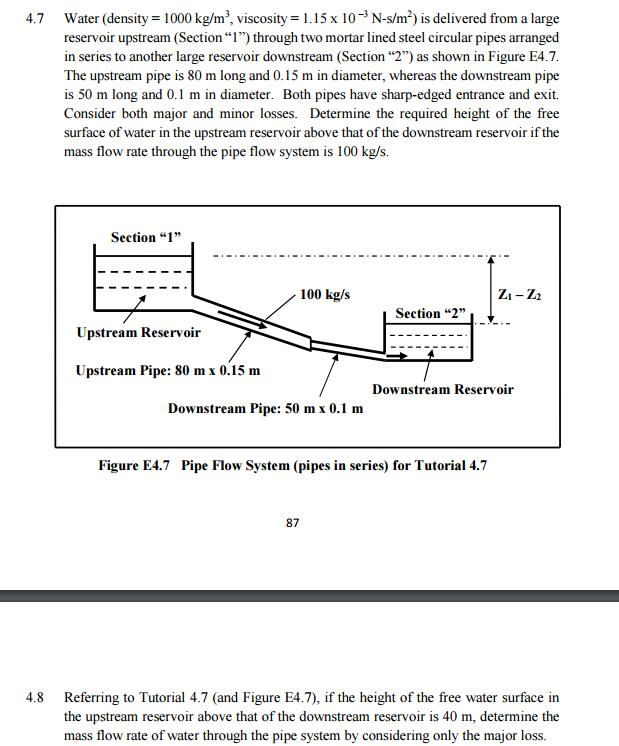Water (density = 1000 kg/m3, viscosity = 1.15 x 10-3 N-s/m2) is delivered from a large reservoir upstream (Section"1") through two mortar lined steel circular pipes arranged in series to another large reservoir downstream (Section "2") as shown in Figure E4.7 The upstream pipe is 80 m long and 0.15 m in diameter, whereas the downstream pipe is 50 m long and 0.1 m in diameter. Both pipes have sharp-edged entrance and exit. Consider both major and minor losses. Determine...

• ### Water flows from one large reservoir to another via a pipeline which is 0.9 m in...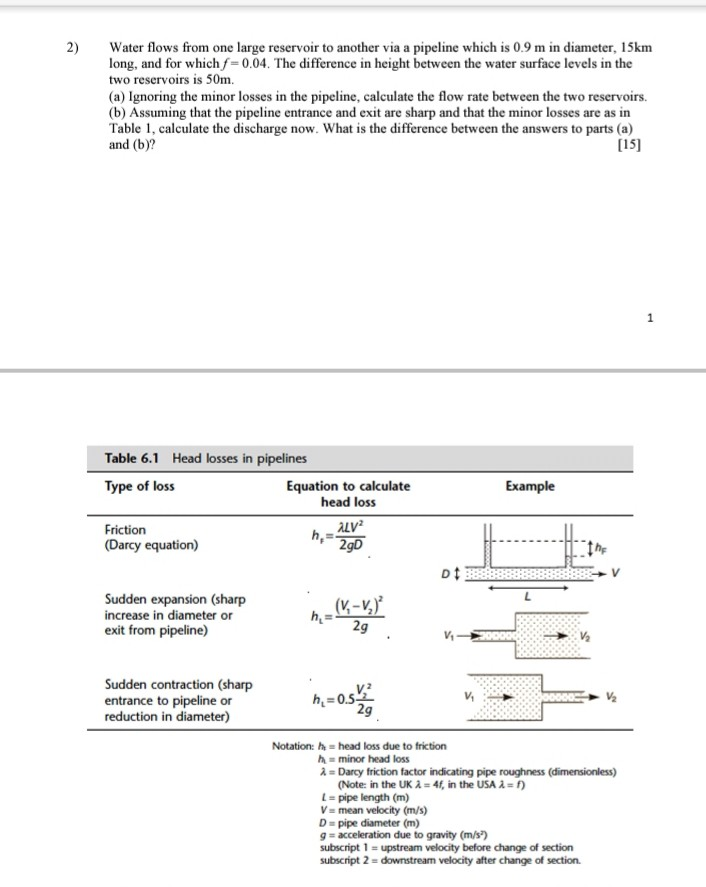Water flows from one large reservoir to another via a pipeline which is 0.9 m in diameter, 15km long, and for which f-0.04. The difference in height between the water surface levels in the two reservoirs is 50m. (a) Ignoring the minor losses in the pipeline, calculate the flow rate between the two reservoirs. (b) Assuming that the pipeline entrance and exit are sharp and that the minor losses are as in Table 1, calculate the discharge now. What is...

• ### 6 10 11 13 14 15 16 Water flows from left reservoir to right reservoir in...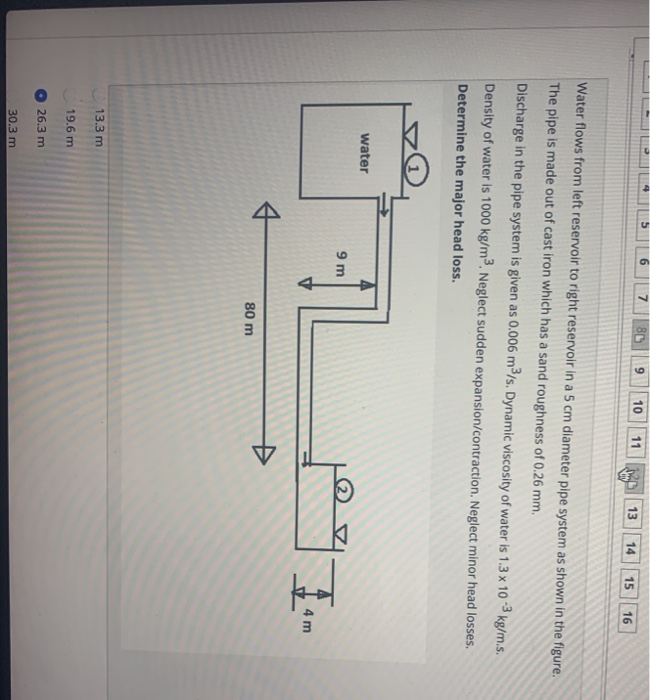6 10 11 13 14 15 16 Water flows from left reservoir to right reservoir in a 5 cm diameter pipe system as shown in the figure. The pipe is made out of cast iron which has a sand roughness of 0.26 mm. Discharge in the pipe system is given as 0.006 m3/s. Dynamic viscosity of water is 1.3 x 10 -3 kg/m.s. Density of water is 1000 kg/m3. Neglect sudden expansion/contraction. Neglect minor head losses. Determine the major head...

• ### Question 3 s points Save Angup Water flows from left reservoir to right reservoir in a...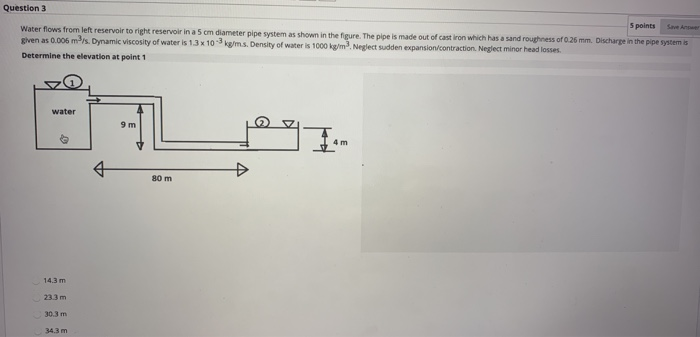Question 3 s points Save Angup Water flows from left reservoir to right reservoir in a 5 cm diameter pipe system as shown in the figure. The pipe is made out of cast iron which has a sand roughness of 0.26 mm. Discharge in the pipe system is given as 0.006 m/s. Dynamic viscosity of water is 13 x 103 kg/m.s. Density of water is 1000 kg/m2. Neglect sudden expansion/contraction. Neglect minor head losses. Determine the elevation at point 1...

• ### 35. (a) A reservoir A discharges water to a lower reservoir B through a 3600 m long pipe of diameter 600 mm. The flow i...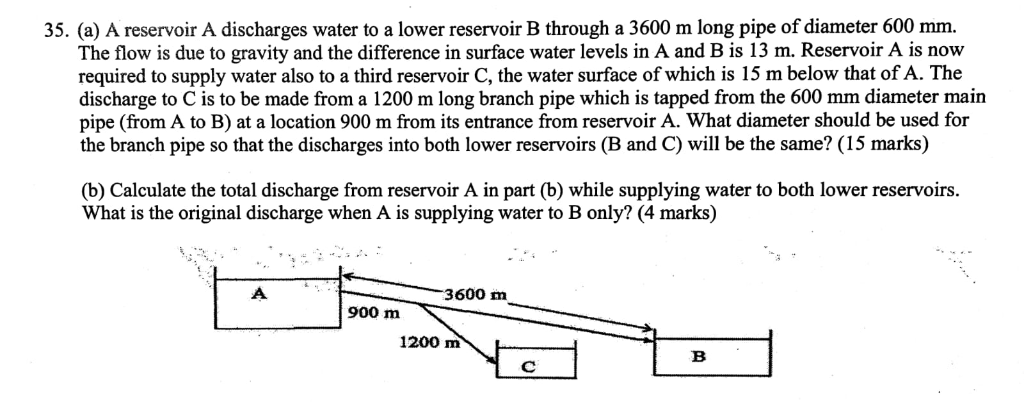35. (a) A reservoir A discharges water to a lower reservoir B through a 3600 m long pipe of diameter 600 mm. The flow is due to gravity and the difference in surface water levels in A and B is 13 m. Reservoir A is now required to supply water also to a third reservoir C, the water surface of which is 15 m below that of A. The discharge to C is to be made from a 1200 m...

• ### Water is to be withdrawn from a 3-m-high water reservoir by drilling a 1.5-cm- diameter hole...

Water is to be withdrawn from a 3-m-high water reservoir by drilling a 1.5-cm- diameter hole in the center of the bottom surface. Disregarding the effect of the kinetic energy correction factor, determine the flow rate of water through the hole for a) a sharp edged entrance followed by threaded 90° bend and then a 3.5m length of wrought iron pipe and finally a fully open Globe Valve b) redo a) for the ideal case with no viscous losses

• ### Please show full working so I can understand how to do each step. Thank you in advance! Two reservoirs with their water...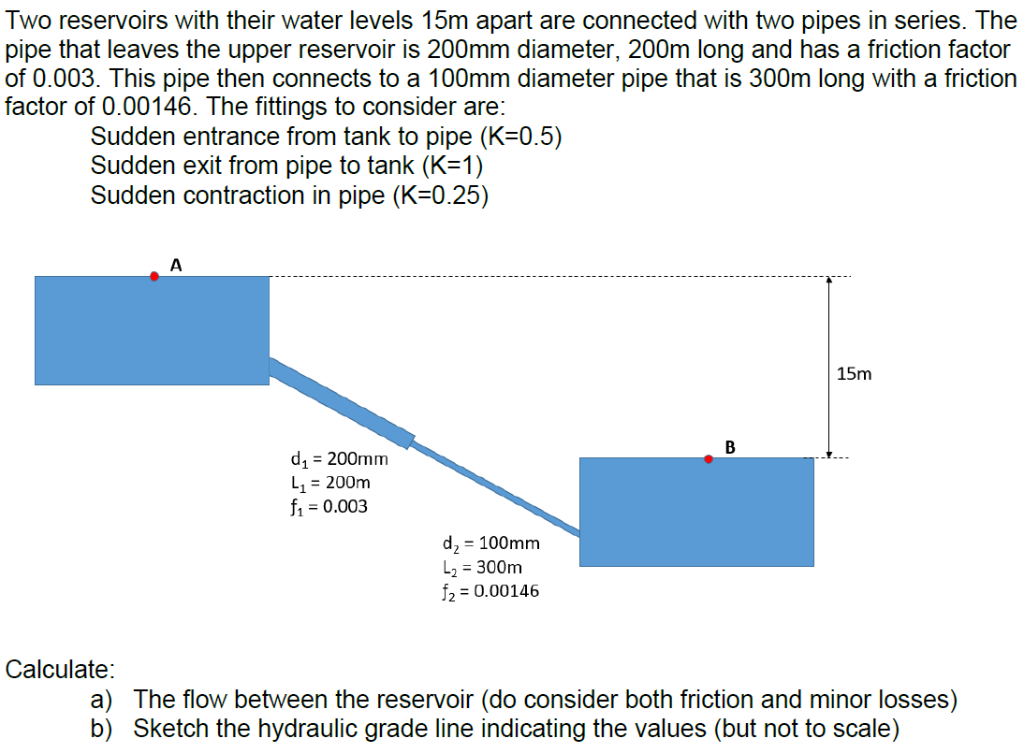Please show full working so I can understand how to do each step. Thank you in advance! Two reservoirs with their water levels 15m apart are connected with two pipes in series. The pipe that leaves the upper reservoir is 200mm diameter, 200m long and has a friction factor of 0.003. This pipe then connects to a 100mm diameter pipe that is 300m long with a friction factor of 0.00146. The fittings to consider are: Sudden entrance from tank to...